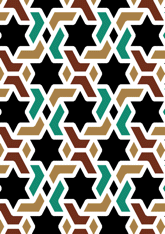data189/IND0428

## Geometry

• The symmetry group of the tiling is 2222 (p2).
• All the internal angles of the constituent polygons are a multiple of 60°.
• Contains two regular two-pointed star polygons with vertex angle of 60°.
• Contains two regular 6-pointed star polygons with vertex angle of 60°.
• There are no non-regular reflective pairs and one tile with no mirror image.
• The tiling does not satisfy the two-colour condition.
• The tiling is not edge-to-edge.
• As drawn, contains about 149 polygons.

## References

Publications referenced: